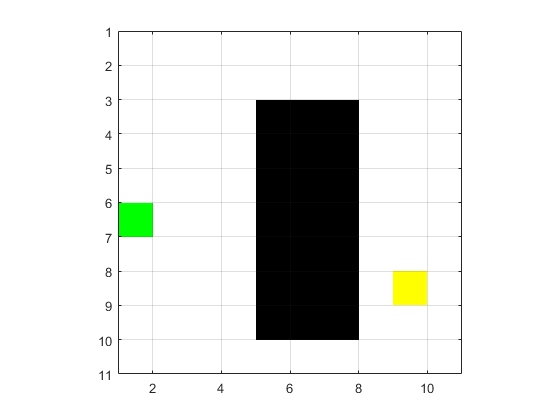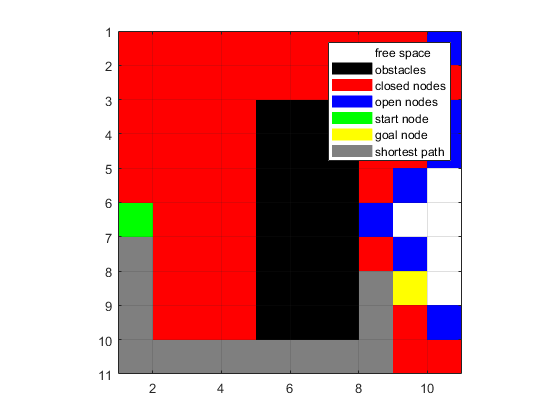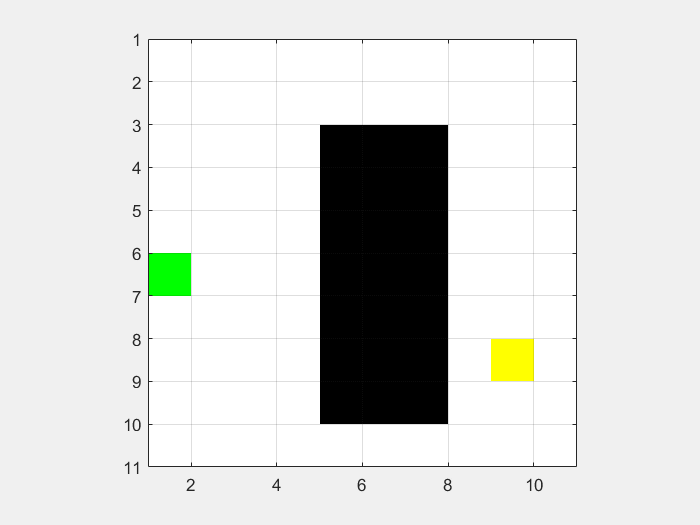Author: Yash Bansod
Date: 15th September 2017
Brief: This demonstrates the Dijkstra path planning algorithm
GitHub: https://github.com/YashBansod

## Initialize the maps, color maps, start points and destination points

``````input_map = false(10);              % Create an Input Map
input_map (3:9, 5:7) = 1;           % Add an obstacle
start_coords = [6, 1];              % Save the location of start coordinate
dest_coords = [8, 9];               % Save location of destination coordinate
cmap = [1   1   1;                  % Create a color map
0   0   0;
1   0   0;
0   0   1;
0   1   0;
1   1   0;
0.5 0.5 0.5];
colormap(cmap);                     % Sets the colormap for the current figure
[nrows, ncols] = size(input_map);   % Save the size of the input_map

map = zeros(nrows,ncols);           % Create map to save the states of each grid cell
map(~input_map) = 1;                % Mark free cells on map
map(input_map) = 2;                 % Mark obstacle cells on map
start_node = sub2ind(size(map), start_coords(1), start_coords(2));      % Generate linear indices of start node
dest_node = sub2ind(size(map), dest_coords(1), dest_coords(2));         % Generate linear indices of dest node
map(start_node) = 5;                % Mark start node on map
map(dest_node) = 6;                 % Mark destination node on map

% Initialize distance from start array to inifinity
distanceFromStart = Inf(nrows,ncols);

% Create a map that holds the index of its parent for each grid cell
parent = zeros(nrows, ncols);

distanceFromStart(start_node) = 0;  % distance of start node is zero

image(1.5, 1.5, map);
grid on;                        % Display grid lines
axis image;                     % Set axis limits
drawnow;                        % Update figure

drawMapEveryTime = true;            % To see how nodes expand on the grid
``````## Process the map to update the parent information and distance from start

``````while true                              % Create an infinite loop
map(start_node) = 5;                % Mark start node on map
map(dest_node) = 6;                 % Mark destination node on map

if (drawMapEveryTime)
image(1.5, 1.5, map);
grid on;                        % Display grid lines
axis image;                     % Set axis limits
drawnow;                        % Update figure
end

% Find the node with the minimum distance
[min_dist, current] = min(distanceFromStart(:));
% Compute row, column coordinates of current node from linear index
[i, j] = ind2sub(size(distanceFromStart), current);

% Create an exit condition for the infinite loop to end
if ((current == dest_node) || isinf(min_dist)) break
end

% Update distance value of element right of current element
if (i+1 <= nrows && distanceFromStart(i+1, j) > min_dist + 1)
if (parent(i+1, j) == 0 && input_map(i+1,j)~=1 && parent(current)~= sub2ind(size(map), i+1, j))
distanceFromStart(i+1, j) = min_dist + 1;
map(sub2ind(size(map), i+1, j)) = 4;    % Mark the neighbour of current as processing
parent(i+1, j)= current;
end
end

% Update distance value of element left of current element
if (i-1 >= 1 && distanceFromStart(i-1, j) > min_dist + 1)
if (parent(i-1, j) == 0 && input_map(i-1,j)~=1 && parent(current)~= sub2ind(size(map), i-1, j))
distanceFromStart(i-1, j) = min_dist + 1;
map(sub2ind(size(map), i-1, j)) = 4;    % Mark the neighbour of current as processing
parent(i-1, j)= current;
end
end

% Update distance value of element top of current element
if (j-1 >= 1 && distanceFromStart(i, j-1) > min_dist + 1)
if (parent(i, j-1) == 0 && input_map(i,j-1)~=1 && parent(current)~= sub2ind(size(map), i, j-1))
distanceFromStart(i, j-1) = min_dist + 1;
map(sub2ind(size(map), i, j-1)) = 4;    % Mark the neighbour of current as processing
parent(i, j-1)= current;
end
end

% Update distance value of element bottom of current element
if (j+1 <= ncols && distanceFromStart(i, j+1) > min_dist + 1)
if (parent(i, j+1) == 0 && input_map(i,j+1)~=1 && parent(current)~= sub2ind(size(map), i, j+1))
distanceFromStart(i, j+1) = min_dist + 1;
map(sub2ind(size(map), i, j+1)) = 4;    % Mark the neighbour of current as processing
parent(i, j+1)= current;
end
end

distanceFromStart(current) = -log(0);   % change the distance of current from start as infinity
map(current) = 3;                       % mark the current point as processed
end
````````````if (isinf(distanceFromStart(dest_node))) route = [];    % if distance to destination node is infinity
else route = [dest_node];                               % else backtrace the route from destination node
while (parent(route(1)) ~= 0)                       % check front of route for start node
route = [parent(route(1)), route];              % add parent of current node to front of route
end

for k = 2:length(route) - 1         % To visualize the map and the path
map(route(k)) = 7;
pause(0.001);                   % Pause the code for a while
image(1.5, 1.5, map);
grid on;                        % Display grid lines
axis image;                     % Set axis lengths
end
end

% Add legends to the colormapped image
hold on;
for K = 1:7
hidden_h(K) = surf(zeros(2, 2), 'edgecolor', 'none', ...
'facecolor', cmap(K, :));
end
hold off

uistack(hidden_h, 'bottom');
legend(hidden_h, {'free space', 'obstacles', 'closed nodes', ...
'open nodes', 'start node', 'goal node', 'shortest path'} )
``````## Results